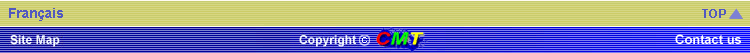# Basic Math Skills

## VOLUMESsquare = s2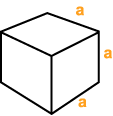cube = a3rectangle = b*h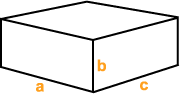rectangular prism = a*b*c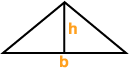triangle = 1/2*b*h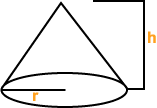cone = 1/3*p*r2*h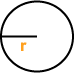circle = p*r2

## ALGEBRA

Additive Identity : a + 0 = a

Additive Inverse : a + (-a) = 0

Associative of Addition: (a+b) + c = a + (b+c)

Commutative of Addition: a + b = b + a

Definition of Subtraction: a - b = a + (-b)

Multiplicative Identity: a * 1 = a

Multiplicative Inverse: a * (1/a) = 1 , where a does not = 0

Associative of Multiplication: (a*b) * c = a * (b*c)

Commutative of Multiplication: a * b = b * a

Distributative Law : a (b+c) = ab + ac

Definition of Division : a / b = a (1/b)